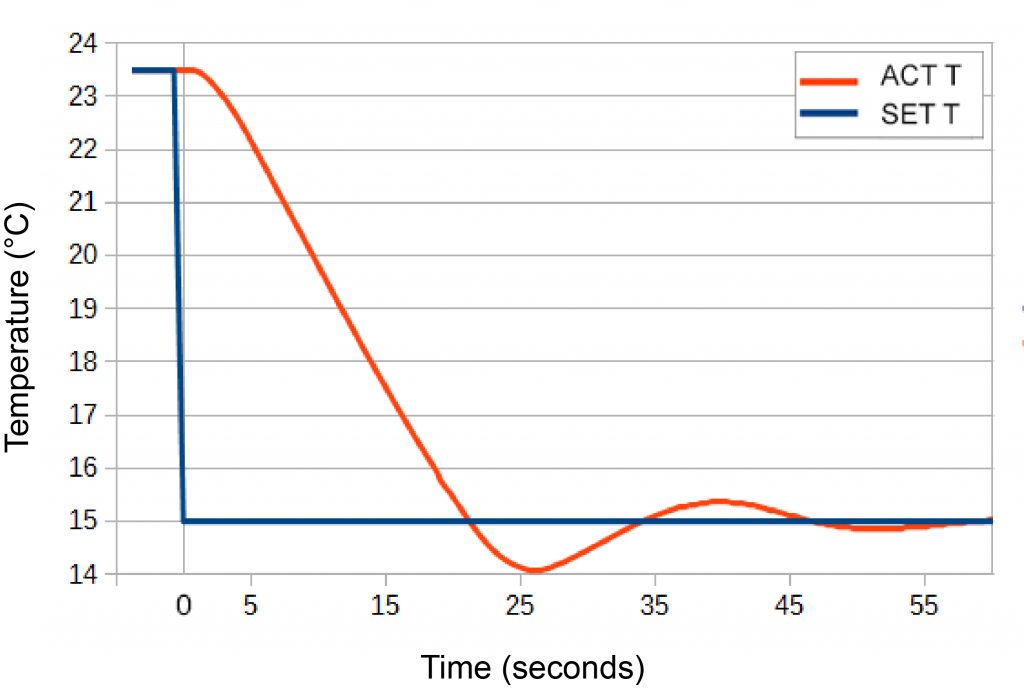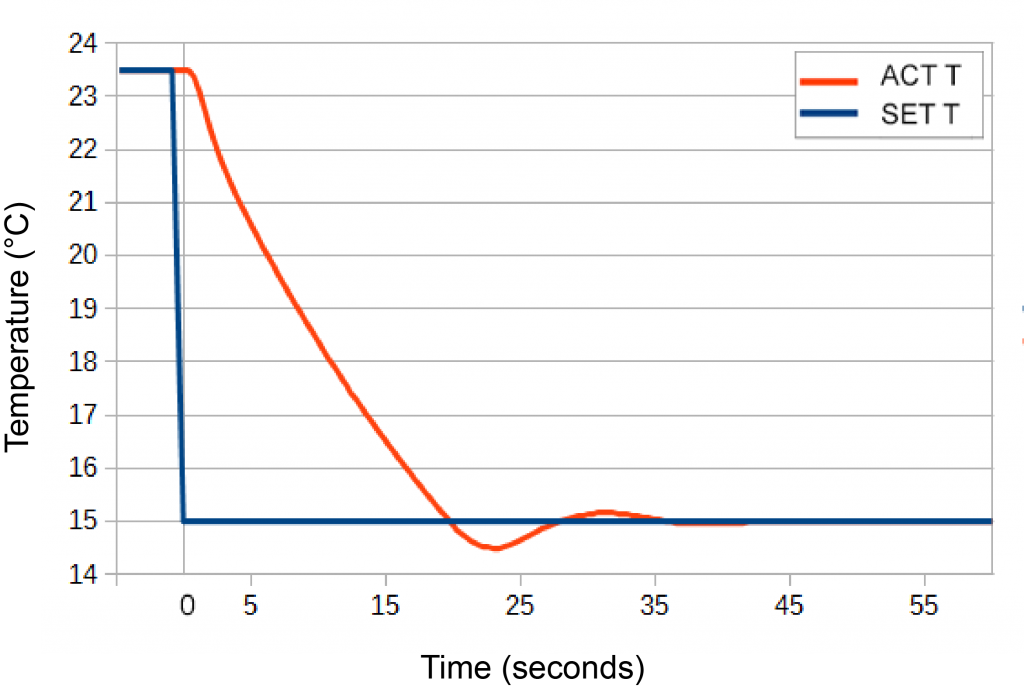LFI3751 ANALOG
LFI3751 DIGITAL
TC5 LAB
TC10 LAB
TC15 LAB

## Question

What effect does the D term have on system response?

The D term can amplify system noise, so we wanted to see its affect on time to temperature. To demonstrate the differences, we compared the 23.5°C to 15°C step response tests with D term OFF (Figure 1) and with a load-optimized D term (Figure 2).

To illustrate the differences, we used a 3 A load with a 10 kΩ thermistor while characterizing IntelliTune® performance.Figure 1. Tuned, 57.1 seconds to stable temperature First crossing of setpoint: 21.3 seconds P=8.214; I=0.685; D=OFF Setpoint ResponseFigure 2. Tuned, 39.9 seconds to stable temperature First crossing of setpoint: 19.9 seconds P=14.081; I=1.408; D=1.594 Setpoint Response

Turning the D term off significantly increased overshoot and extended time to temperature. The D term roughly acts as a brake to offset the accelerator (P).